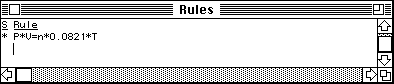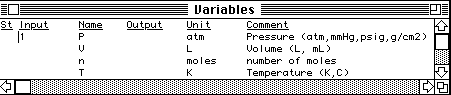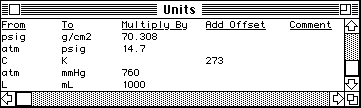## Solving problems with TK Solver Plus

TK Solver Plus is a tool for numerically solving sets of equations, even when you can't (or don't want to) get an explicit algebraic solution for the problem. All you do is list all the equations that relate to the problem, then specify the values of any variables that are known. The program then calculates the values of any unknown variable that can be calculated according to the rules of algebra, automatically solving the equations and making substitutions as needed. (TK Solver Plus provides numerical answers only; it does not provide symbolic solutions and does not "show its work", that is, tell you what particular substitutions it took to arrive at an answer.)

TK Solver Plus lets you explore the behavior of mathematically inconvenient systems (such as a Van der Walls real gas) and crank out the answers to numeric word problems much more simply than by using a hand calculator, writing programs, or using a spreadsheet. The big advantage over a spreadsheet is that you don't need to have an explicit algebraic solution. Moreover, one set of equations (called a model in TK-talk) can be used to solve many variations on one type of problem, because any variable can be a known or an unknown.

TK Solver can also handle unit conversions. Once you have defined a set of unit conversion factors, you can enter known variables in any of those units and ask for unknowns to be displayed in any of those units.

For problems that can not be solved by direct substitution, the program has an terative solver that can solve just about any consistent set of equations (albeit more slowly than the direct solver). You enter a first guess for one (or more) variables.

### How to develop a TK Solver Plus model

In this section, we will develop a simple model to solve problems relating to the ideal gas las, i.e. PV=nRT. This model will have only one equation and 4 variables (R being a constant)

When you launch TK Solver Plus, you'll see a column of icons on the left of the screen. Double-click on the Rules icon to bring up the Rule sheet.1. Type all the equations that relate to the problem on the Rule Sheet in standard "computer algebra" notation and press RETURN after each equation. In this case there is only one equation, e.g. P*V=n*0.0821*T. Be sure to use unique variable names for each variable. Also make sure that the equations are based on a consistent set of physical units; that is, if one equation is written for L in liters, the all the equations must be written for L in liters.

As you enter the equations, a list of the variables is automatically generated on the Variable Sheet. To see the Variable Sheet, double-click on the Variables icon.2. For each variable in the Variable Sheet, type in the units for which the rules hold in the Unit column. In this case, since we used a value of 0.082 liter-atm/K-mole for R, L must be in Liters, P in atmospheres, T in Kelvins, and n in number of moles. You can make up your own set of abbreviations for units, but you must be internally consistent.

3. (Optional) Double-click on the Units icon to bring up the Units sheet. Type in any alternative units and the appropriate conversion factors. Your abbreviations for units must be consistent with what you typed in the units column of the Variable sheet.4. (Optional) Briefly describe each variable in a note added to the Comment column on the Variable Sheet, including a list of any alternative units for which you have defined conversion factors in the Unit Sheet.

### How to use a TK Solver model

1. For each variable whose value is known, type in first its units in the Unit column (if different than what is already there) and then its value in the Input column. (You'll find that it's best to enter the units first and then the value. The units must be one of the ones defined on the Unit sheet). Leave the Input column blank for unknown variables.

2. Type = to solve the model. Any unknown quantities that can be calculated from the known quantities by the direct solver will be computed and displayed in the Output column in the units specified in the Unit column. You can change the units to other alternatives if desired.

3. If that does not seem to work, try the iterative solver. Type a G in the St column of one of the unknown variables in the Variable sheet and enter a reasonable estimate of its value into the Input column. This defines that variable as the guess variable. Type = to start the iterative solver. The solution will usually converge in a few iterations. In difficult cases you might have to try different variables as the guess variable or different values for the first guess value.

4. Save and Print the model in the usual way. Only the active sheet is printed. You can elect to "print" the model "to file", which is TK-talk for saving the model as an ASCII text file.

5. You can load a saved model from the disk with the Open command in the File menu, as usual. The program then displays a dialog box that asks you: Merge file with current model, or reset the current model first? Ordinarily, you'll click on Reset, which loads the new model in place the previous one.

6. There is actually much more to TK Solver Plus. It can plot graphs, handle lists of input and output variables, user-defined functions, etc. See the manual.

References: There is an introductory tutorial in the manual. On-line help is available from About TK Solver in the Apple menu. (The TK Solver folder contains also some tutorials in the form of Hypercard stacks; double-click on them to run then or open them with HyperDA.)

There are two books that describe chemistry applications of the program: Applications of TK Solver Plus in Chemical Equilibrium and Chemical Analysis, by Alan Smith of Drexel Univ (McGraw-Hill, 1990), and General Chemistry with TK Solver, by Don Peterson, U. of San Diego.